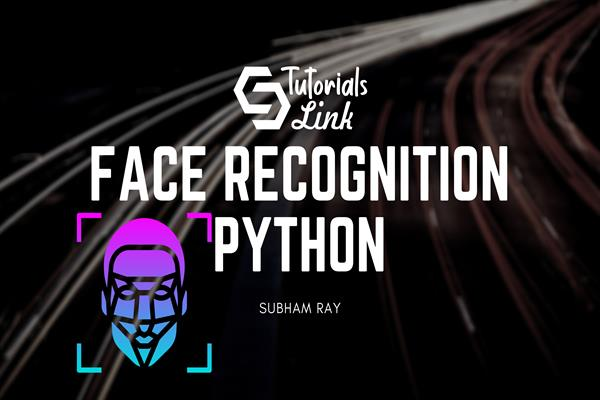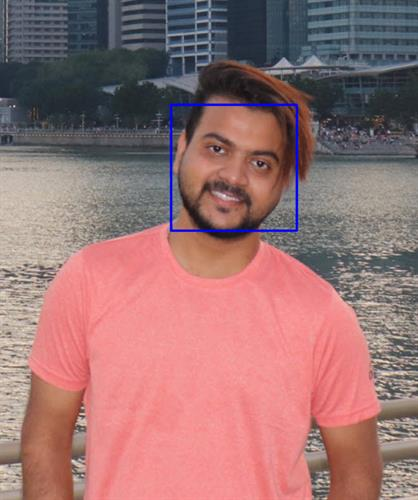Loading, please wait...# How to build Face Recognition in Python from scratch | Python Tutorial

Oct 27, 2020 Python, 3905 Views
Face Recognition in Python from Scratch

## Face recognition in Python

Introduction

Python programming language is a high-level programming language with the support of built-in high dynamic semantics which adds functionality to codes with easiness and more of function derived attributes. In talking to the same in this article we will build a face recognition model in python from very scratch i.e, from very installation and setting up the environment and explaining each and every line of code.

Steps

If this is your first time working with Opencv then you should install or download using the following command :

``pip install opencv-python``

Upon successful downloading open your IDE here I am using a jupyter notebook to do my code. The next phase is to import the library so here, I import the library using the following code

``````#import library
import cv2``````

The very next phase is to import the picture which you will test for the purpose of model testing, we implement using the following command

``````#Input image

The next step is to load the facial cascade and we implement it using cascade classifier using the following code

``````# Load the face cascade
``````

Converting the image into grayscale is the next process when we implement the code to convert it to grayscale as below:

``````grayscale = cv2.cvtColor(image, cv2.COLOR_BGR2GRAY)
``````

Detecting faces in the next phase where we detect the faces in the image

``faces = facial.detectMultiScale(grayscale, 1.1, 4)``

After detecting the face, we need to implement the rectangle around the face, so we do it by the following code

``````for (x, y, w, h) in faces:
cv2.rectangle(image, (x, y), (x+w, y+h), (255, 0, 0), 2)``````

And the final step is to show the output with the wait key

``````cv2.imshow('image', image)
cv2.waitKey()``````

The final output in my experiment is :The model works well and it can be optimized and more functionality can be added. It was an article that boosted you to understand the working of a face recognition model from scratch.